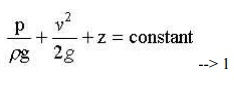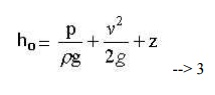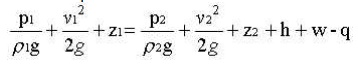Home | | Fluid Mechanics and Machinery | Concept of Control Volume

# Concept of Control Volume

A specified large number of fluid and thermal devices have mass flow in and out of a system called as control volume.

CONCEPT OF CONTROL VOLUME

A specified large number of fluid and thermal devices have mass flow in and out of a system called as control volume.

1.CONTINUITY EQUATION

Concepts

The continuity equation is governed from the principle of conservation of mass.It states that the mass of fluid flowing through the pipe at the cross-section remains constants,if there is no fluid is added or removed from the pipe.

Let us make the mass balance for a fluid element as shown below: (an open-faced cube)This is the continuity equation for every point in a fluid flow whether steady or unsteady , compressible or incompressible.

For steady, incompressible flow, the density is constant and the equation simplifies toFor two dimensional incompressible flow this will simplify still further to2 EULER'S EQUATION OF MOTIONThis is known as Euler's equation, giving, in differential form the relationship between p, vr and elevation z, along a streamline for steady flow.

3 BERNOULLI EQUATION

Concepts

Bernoulli’s Equation relates velocity, pressure and elevation changes of a fluid in motion. It may be stated as follows “ In an ideal incompressible fluid when the flow is steady and

continuous the sum of pressure energy, kinetic energy and potential energy is constant along streamline”This is the basic from of Bernoulli equation for steady incompressible inviscid flows. It may be written for any two points 1 and 2 on the same streamline asThe contstant of Bernoulli equation, can be named as total head (ho) has different values on different streamlines.The total head may be regarded as the sum of the piezometric head h* = p/rg + z and the kinetic head v2/2g.

Bernoullie equation is arrived from the following assumptions:

1.     Steady flow - common assumption applicable to many flows.

2.     Incompressible flow - acceptable if the flow Mach number is less than 0.3.

3.     Frictionless flow - very restrictive; solid walls introduce friction effects.

4.     Valid for flow along a single streamline; i.e., different streamlines may have different ho.

5.     No shaft work - no pump or turbines on the streamline.

6.     No transfer of heat - either added or removed.

Range of validity of the Bernoulli Equation:

Bernoulli equation is valid along any streamline in any steady, inviscid, incompressible flow. There are no restrictions on the shape of the streamline or on the geometry of the overall flow. The equation is valid for flow in one, two or three dimensions.

Modifications on Bernoulli equation:

Bernoulli equation can be corrected and used in the following form for real cases.APPLICATIONS

1.Venturimeter.

2.Orificemeter

3.Pitot Tube

Study Material, Lecturing Notes, Assignment, Reference, Wiki description explanation, brief detail
Mechanical : Fluid Mechanics And Machinery : Fluid Properties and Flow Characteristics : Concept of Control Volume |

Related Topics